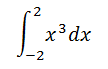# definite integral of x^3

## Recommended Posts

when I tried to do:I came to the answer of 0 and I know this is not right.

I do remember that you had to split the problem up and then integrate those or something like that.

Could someone tell me the answer with their method.

##### Share on other sites

Why do you think 0 is incorrect?

If you sketch $x^3$ you might be able to get an intuitive feel for the answer.

##### Share on other sites

What's wrong with the answer 0?

EDIT: That post of mine was less pointless an hour ago when I opened this thread in a new tab and then forgot about it .... .

Edited by timo
##### Share on other sites

It would be helpful to note that the definite integral counts areas below the axis as negative and areas above the axis as positive so the integral between limits may not equal the total area under the curve.

In order to calculate the total area under the curve you do indeed have to split the range of integration and add up the absolute values of all the areas, regardless of sign.

##### Share on other sites

HINT: The fundamental theorem of calculus states that the definite integral of a function over [a,b] is equal to the difference in the anti-derivatives of the function with values respectively as a and b.

##### Share on other sites

The reason why I thought 0 was the wrong answer, was because in a book I read it split the integral up into two different integrals and then added them together.

But, I don't own that book anymore or remember the method very well.

Anyway, I'll just take 0 as the answer.

##### Share on other sites

The reason why I thought 0 was the wrong answer, was because in a book I read it split the integral up into two different integrals and then added them together.

But, I don't own that book anymore or remember the method very well.

Anyway, I'll just take 0 as the answer.

If you want the absolute area, you need to split the integral at x=0. 0 is the result of integrating any odd function over an interval symmetric around 0.

##### Share on other sites

Please tell us exactly what the question was! If it was simply to find $\int_{-2}^2 x^3 dx$ then the answer is, indeed, 0.

But if the question was to find the area of the region bounded by the x-axis and the graph of $x^3$ then you need to do it in two parts because those graphs cross at x= 0. For x> 0, the graph of $y= x^3$ is above the graph of y= 0 so the area is $\int_0^2 (x^3- 0) dx$. For x< 0 the graph of $y= x^3$ is below the graph of $y= 0$ so the area is $\int_{-2}^0 (0- x^3) dx= -\int_{-2}^0 x^3 dx$.

Edited by Country Boy

## Create an account

Register a new account